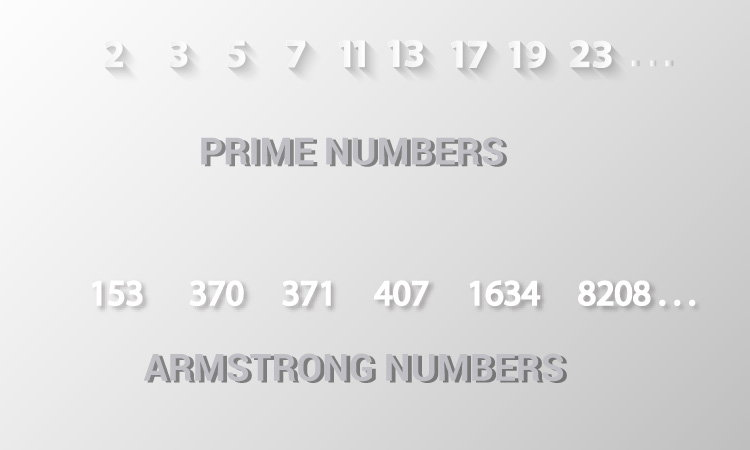# C Program to Check Prime or Armstrong Number Using User-defined Function

Example to check whether an integer is a prime number or an Armstrong or both by creating two separate functions.To understand this example, you should have the knowledge of following C programming topics:

In this program, two user-defined functions `checkPrimeNumber()` and `checkArmstrongNumber()` are created.

The `checkPrimeNumber()` returns 1 if the number entered by the user is a prime number. Similarly, `checkArmstrongNumber()` returns 1 if the number entered by the user is an Armstrong number.

Visit these pages to learn to check whether a number is

## Example: Check Prime and Armstrong Number

``````#include <stdio.h>
#include <math.h>

int checkArmstrongNumber(int n);

int main()
{
int n, flag;

printf("Enter a positive integer: ");
scanf("%d", &n);

// Check prime number
if (flag == 1)
printf("%d is a prime number.\n", n);
else
printf("%d is not a prime number.\n", n);

// Check Armstrong number
flag = checkArmstrongNumber(n);
if (flag == 1)
printf("%d is an Armstrong number.", n);
else
printf("%d is not an Armstrong number.",n);
return 0;
}

{
int i, flag = 1;

for(i=2; i<=n/2; ++i)
{

// condition for non-prime number
if(n%i == 0)
{
flag = 0;
break;
}
}
return flag;
}

int checkArmstrongNumber(int number)
{
int originalNumber, remainder, result = 0, n = 0, flag;

originalNumber = number;

while (originalNumber != 0)
{
originalNumber /= 10;
++n;
}

originalNumber = number;

while (originalNumber != 0)
{
remainder = originalNumber%10;
result += pow(remainder, n);
originalNumber /= 10;
}

// condition for Armstrong number
if(result == number)
flag = 1;
else
flag = 0;

return flag;
}``````

Output

```Enter a positive integer: 407
407 is not a prime number.
407 is an Armstrong number.```